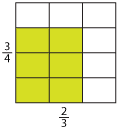Multiplying fractions

How do we multiply two fractions, such as $$\dfrac{2}{3}×\dfrac{3}{4}$$?

We can look at an area model. Take a square of side length 1 and divide it into rectangles by drawing vertical and horizontal lines as shown below.

We divide the height into quarters and the width into thirds. Each rectangle has an area of $$\dfrac{1}{12}$$. If we now shade the region with side lengths $$\dfrac{2}{3}$$ and $$\dfrac{3}{4}$$, we can see that we have an area of $$\dfrac{6}{12}$$. So the area of the shaded region is the product of its sides $$\dfrac{2}{3}×\dfrac{3}{4}$$. This is also equal to 6 lots of $$\dfrac{1}{12}$$.Detailed description

We conclude that $$\dfrac{2}{3}×\dfrac{3}{4}=\dfrac{6}{12}=\dfrac{1}{2}$$. So we can see that the rule for multiplying two fractions is:

Multiply the numerators and multiply the denominators.

This can be written as

$$\dfrac{a}{b}×\dfrac{c}{d}=\dfrac{a×c}{b×d}$$

Simplify if possible.

As before, when we say $$\dfrac{2}{3}$$ of $$\dfrac{3}{4}$$, we mean $$\dfrac{2}{3}×\dfrac{3}{4}$$ .

Example 3

1. $$\dfrac{3}{4}×\dfrac{10}{7}=\dfrac{3×10}{4×7}$$
2. $$\dfrac{1}{6}\ \text{of}\ \dfrac{5}{2}$$

Solution

1. \begin{align}\dfrac{3}{4}×\dfrac{10}{7}&=\dfrac{3×10}{4×7}\\\\ &=\dfrac{30}{28}\\\\ &=\dfrac{15}{14}\\\\ &=1\dfrac{1}{14}\end{align}
2. \begin{align}\dfrac{1}{6}\ \text{of}\ \dfrac{5}{2}&=\dfrac{1×5}{6×2}\\\\ &=\dfrac{5}{12}\end{align}

Cancelling

Again, we can streamline the process by cancelling before multiplying.

Example 4

Evaluate $$\dfrac{2}{3}\ \text{of}\ \dfrac{3}{4}.$$

Solution

\begin{align}\dfrac{2}{3}×\dfrac{3}{4}&=\dfrac{2^1\hspace{-6mm}\color{darkred}{\setminus}}{3\hspace{-4mm}\color{darkred}{\setminus}}×\dfrac{3\hspace{-4mm}\color{darkred}{\setminus}}{4^2\hspace{-6mm}\color{darkred}{\setminus}}\\\\ &=\dfrac{1}{2}\end{align}

Example 5

$$\dfrac{8}{9}×\dfrac{5}{18}×\dfrac{9}{10}$$

Solution

\begin{align}\dfrac{8}{9}×\dfrac{5}{18}×\dfrac{9}{10}&=\dfrac{8^4\hspace{-6mm}\color{darkred}{\setminus}}{9^1\hspace{-6mm}\color{darkred}{\setminus}}×\dfrac{5^1\hspace{-6mm}\color{darkred}{\setminus}}{18}×\dfrac{9^1\hspace{-6mm}\color{darkred}{\setminus}}{10^2\hspace{-6mm}\color{darkred}{\setminus}}\\\\ &=\dfrac{4^2\hspace{-6mm}\color{darkred}{\setminus}}{18^9\hspace{-7mm}\color{darkred}{\setminus}}\\\\ &=\dfrac{2}{9}\end{align}